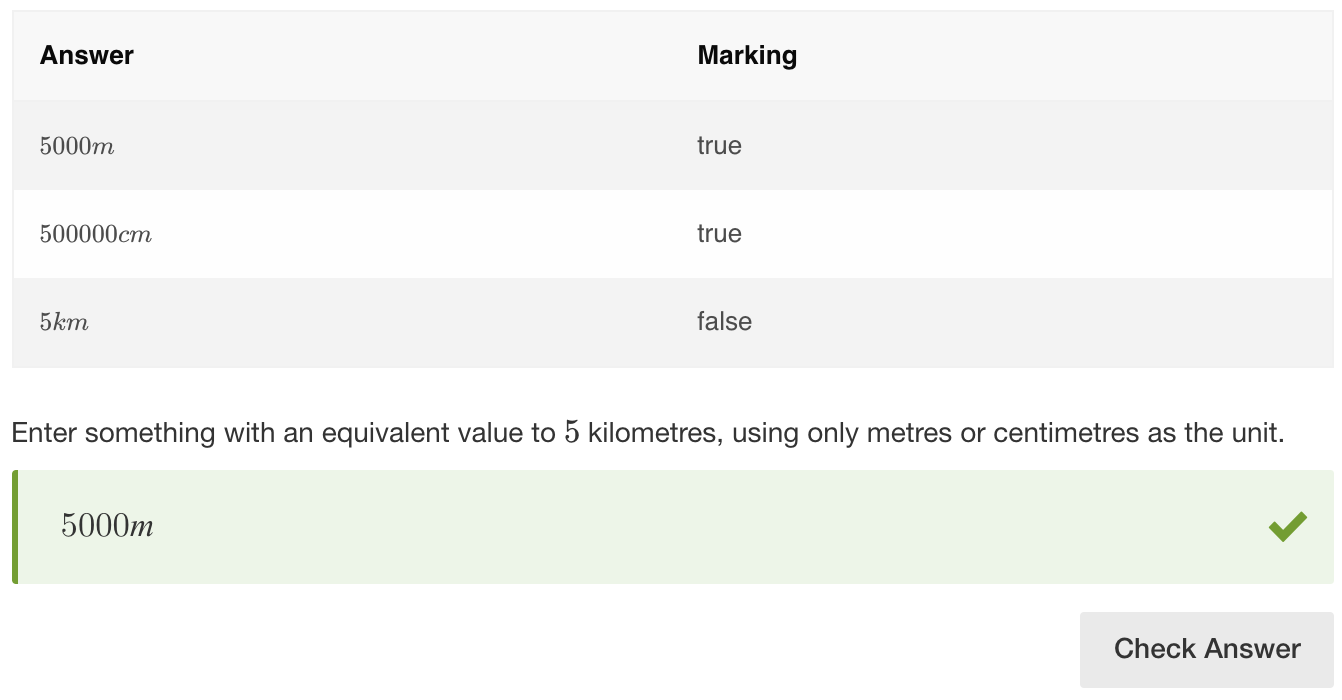# equivValue

## Overview

The equivValue method compares numerical values that may be represented in different ways, such as units of measurement, where 1m = 100cm. equivValue will evaluate the expression to a numerical form for comparison. As long as the value given by the student is mathematically equal to the result the author was expecting, the question will validate as correct.

Although similar to equivSymbolic, the equivValue method cannot be used with variables. It does, however, work with decimals, fractions, and percentages. equivValue is particularly useful if you are using units of measurement. This method can also handle the validation of a range of values, using the plus-minus (±) tolerance symbol.

• Tolerance
A plus-minus (±) value can be added by authors to the value field in the validation area. This means that students can give a response with tolerance that will be deemed as correct. For example, if the valid response set by the author is 10±1.5 the student can respond with any value from 8.5 to 11.5 and get points. Tolerance works only with the the equivValue method.

• Allow decimal marks
Authors can specify what separators can be used by the student. From the thousand Separator drop-down menu, you can select dot, comma, and/or space. The decimal separator menu contains the option for either a dot or a comma. Note that the specified thousand separator and decimal separator cannot be the same, e.g. both dot.

• Significant decimal places
This option specifies the number of significant decimal places used to compare the learner's response and the Author's validation value. Default and the maximum value is 10.

• Ignore text
This refers to LaTeX text only, and when enabled will ignore any LaTeX text the student enters in the response area.

• Compare sides
With this option enabled, when comparing two equations the math engine will compare the corresponding sides of each equation rather than the whole equations.

## Examples

### Example 1 - basic equivValue scoring

As long as the student's answer has the same value as the response set by the author then their response will be correct.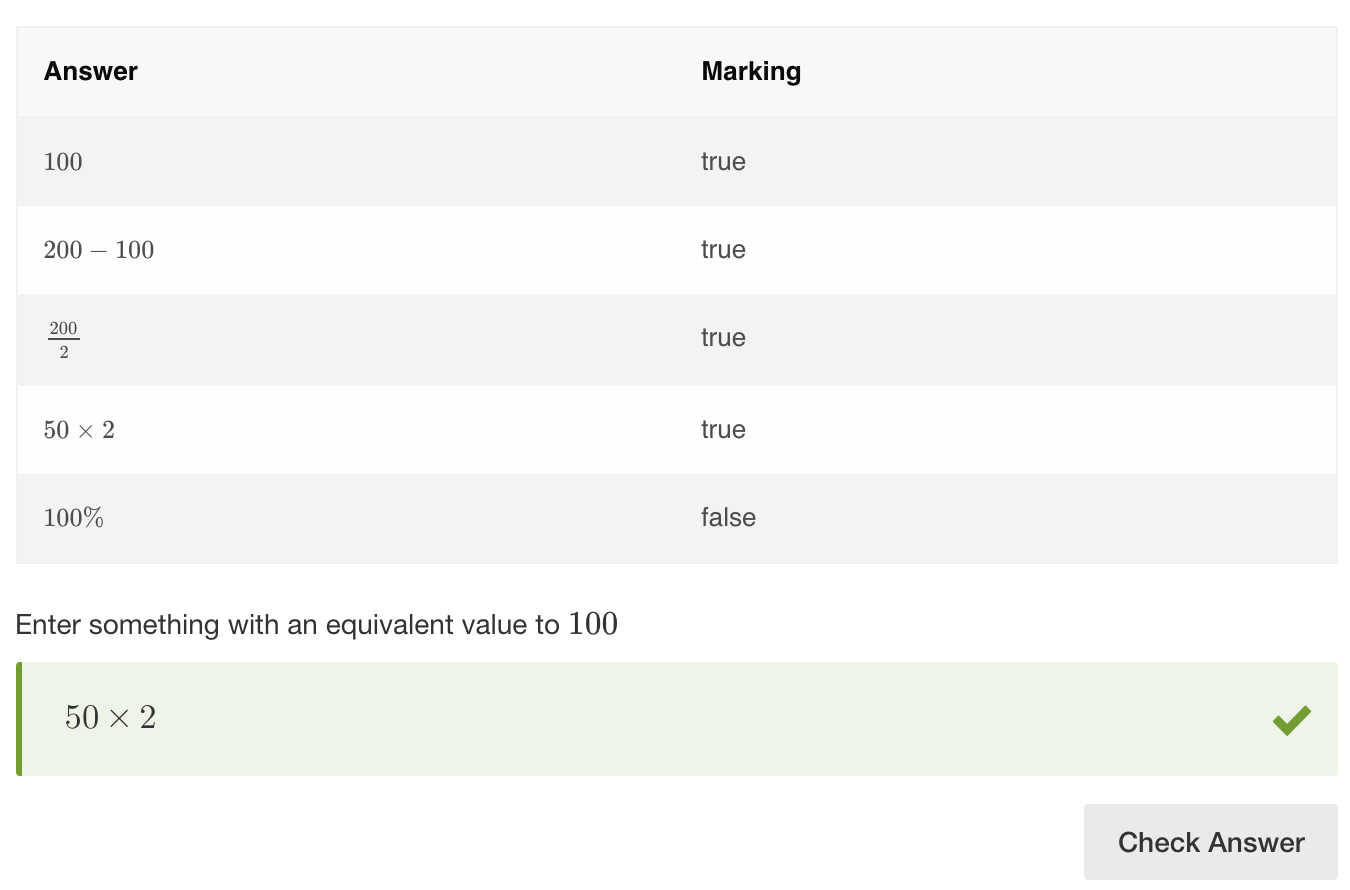### Example 2 - using units

equivValue is very often used with units. In this example, a value given in any applicable unit of measurement that is equal to 1 mile will be correct. US Customary Units must be used.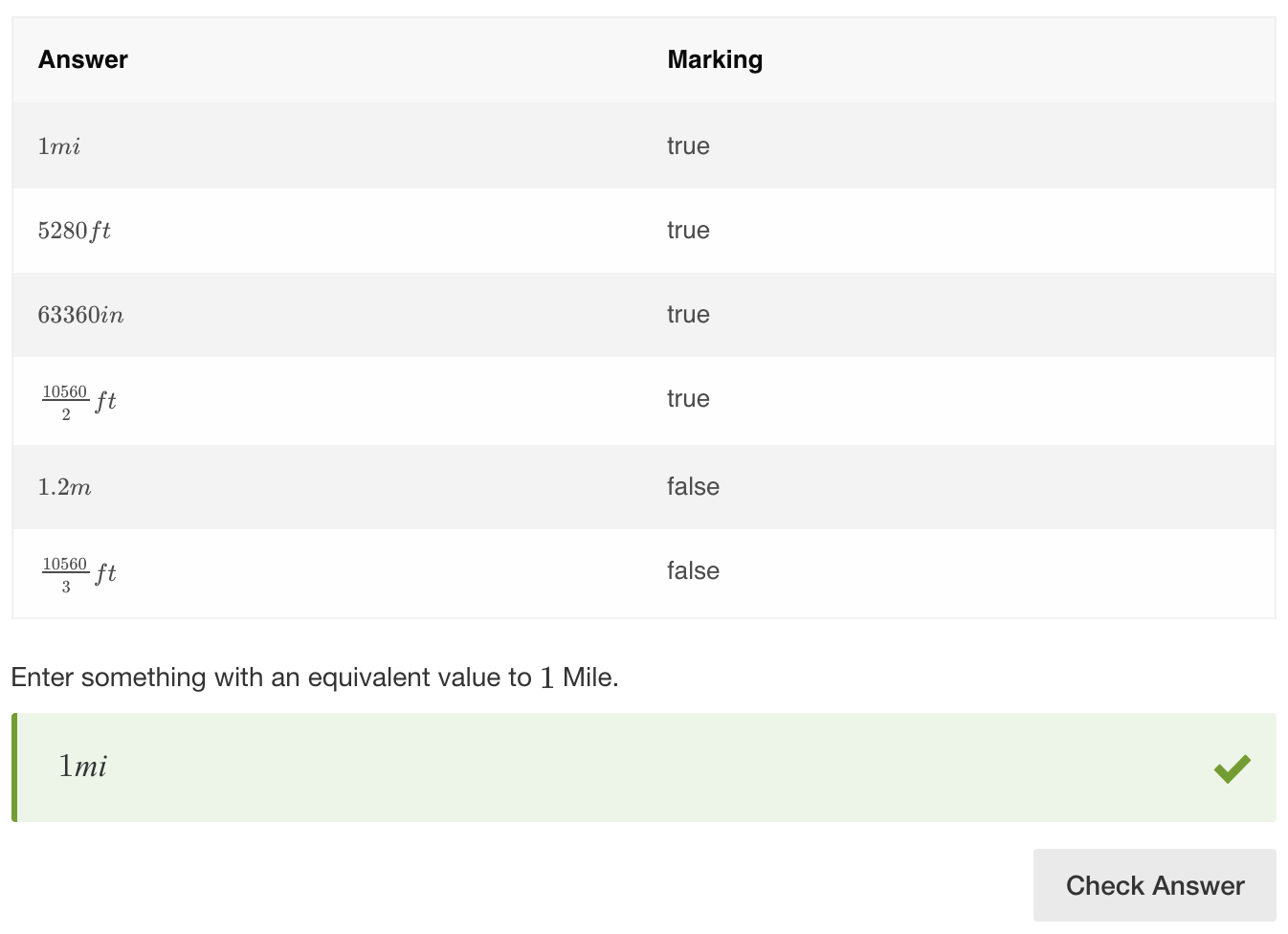### Example 3 - tolerance (plus/minus)

A plus-minus value can be set in validation, so that the correct response is within a range. In this example, a tolerance of 1.6 is applied.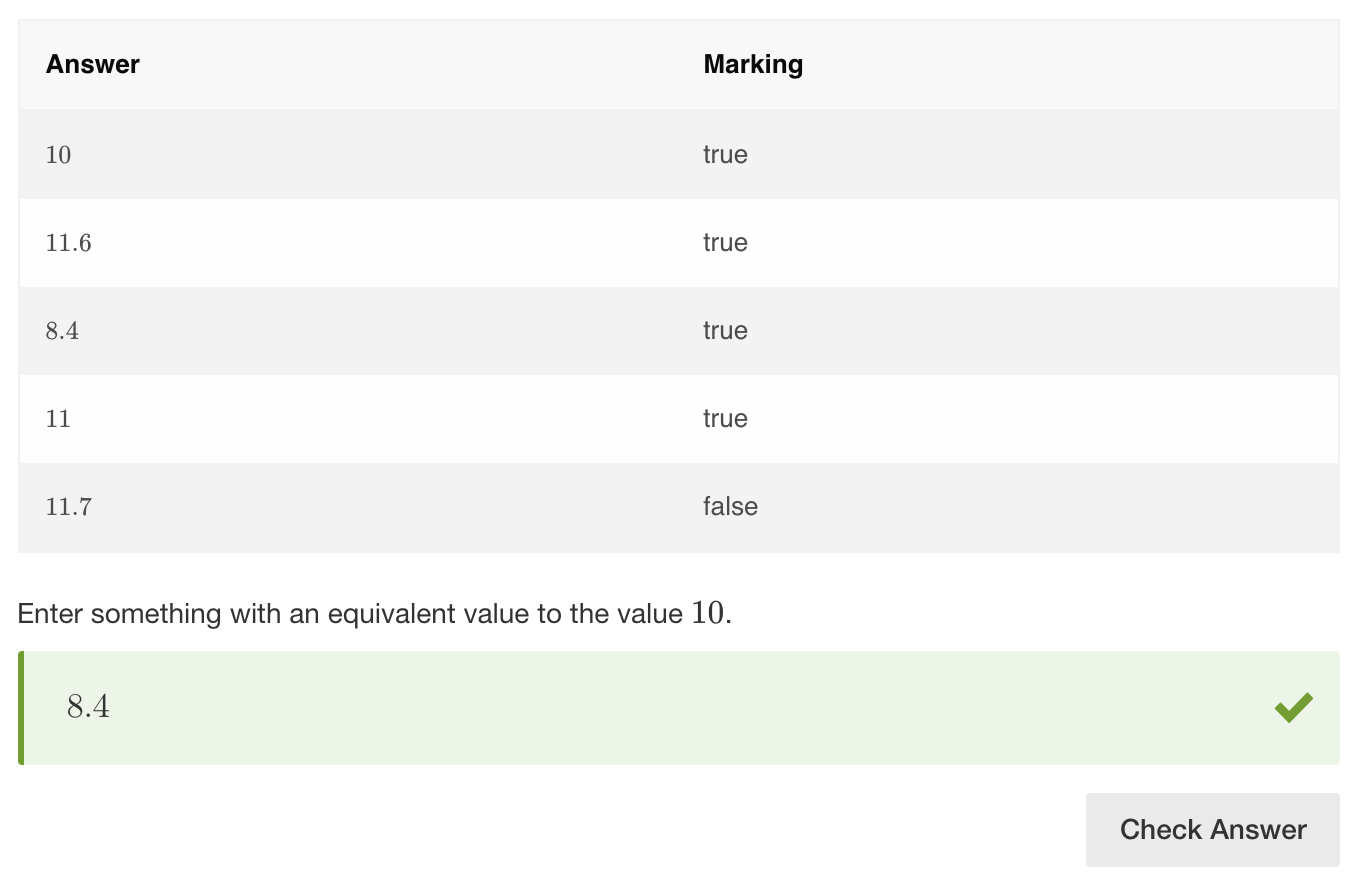### Example 4 - significant decimal places

The significant decimal places field is used to specify the number of significant decimal places the response is rounded to before scoring. In this example, significant decimal places is set to 3. Students will not need to enter all digits after the decimal point for the response to be deemed correct.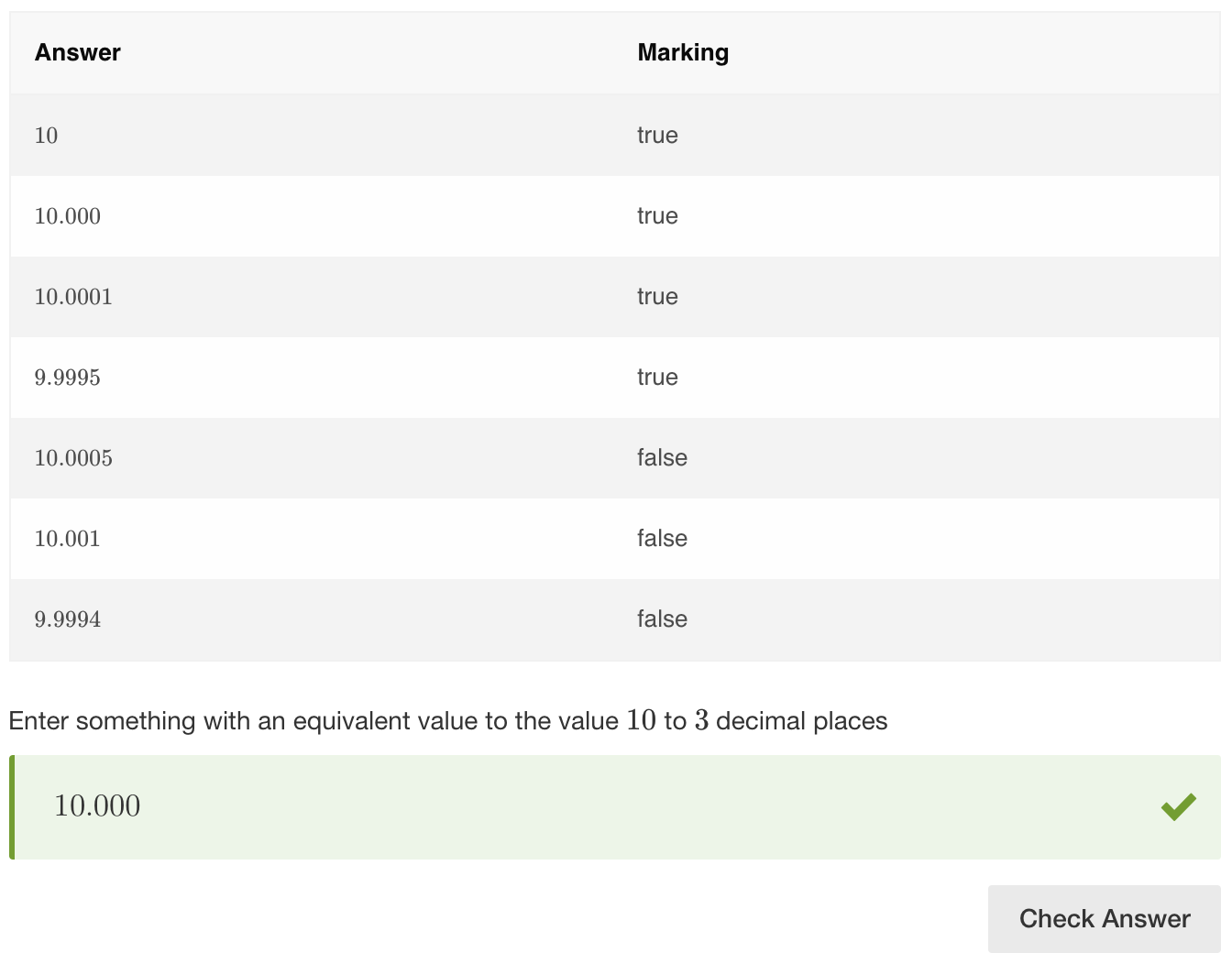### Example 5 - compare sides

In this example students have to enter responses in the empty boxes. A student's response will be considered correct as long as the left side of the equation is mathematically equal to the right side. Validation settings must contain one of the possible correct responses. In this example, it is set to 4+5=9.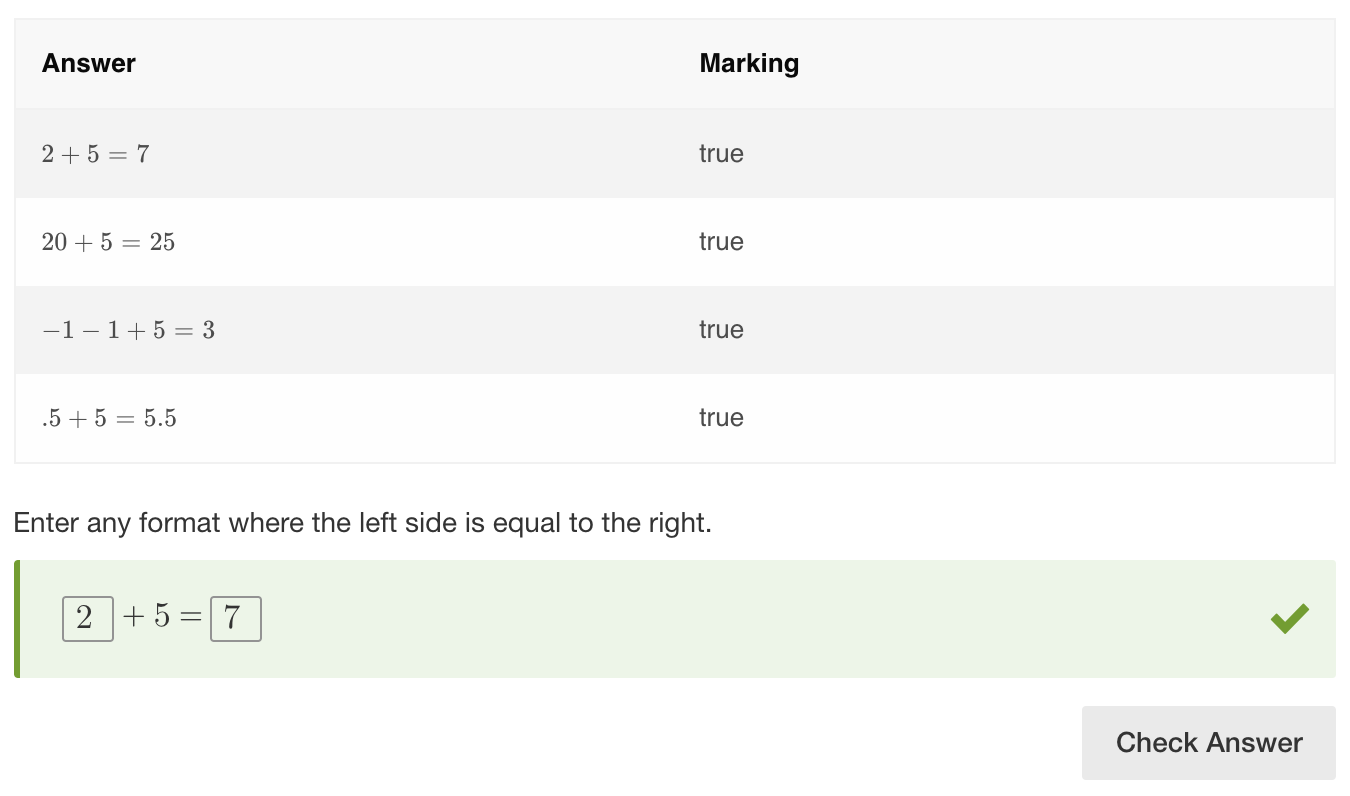## Combining methods

equivValue is often combined with isUnit in order to restrict units that can be accepted as a correct answer.

### Example - equivValue and isUnit

Combining equivValue with isUnit is a good method for narrowing possible responses when it is needed. In this example, the answer should be given in either cm or m. Listing cm and m in isUnit settings ensures that values given in these units of mesurement only will be correct. Also note that even though 5km is set as a valid response in equivValue settings, this answer will be incorrect due to the specified isUnit restrictions.